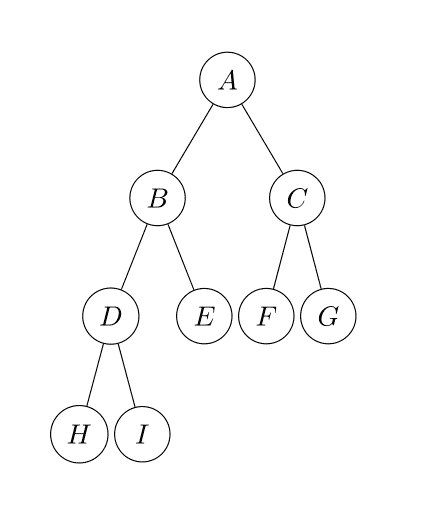## Data structures, Binary trees

So far we had seen linear structures, linked list, queues, stack, arrays, all of them are linear data structures with well defined operations. Trees are our first non-linear data structure and, as the name says, they are data structures that looks like a tree (or an inverted tree).

Each element in a tree is named a node and the first node, the node at the top, is named root, a node can have none or more children and each children has a parent, the “connections” between each node are called edges, nodes without children (with the exception of the root node) are called leaves, the number of edges from the root to a node is called the depth of the tree and the distance between the longest path of a node to the root is called the height of a node, so to know the height of a node or tree we count the number of nodes from that node to the root node (inclusive). In the image we see the node $$D$$ has a depth of 2 and the whole tree has a height of 4, nodes $$H$$, $$I$$, $$E$$, $$F$$ and $$G$$ are leaves.Depending on the maximum number of children per node we have different types of trees, the simplest is a tree where each node has a maximum of 2 children (binary trees) and we will be exploring them extensively in this and upcoming blog posts. There are trees where nodes have up to three children (ternary trees) and even 4 (quaternary) and 8 (octary) trees, in general we usually talk about $$n$$-trees but we will be limiting ourselves to binary trees for now.

A simple way to assemble a binary tree is with a simple structure to hold the data and references to its left and right children, something simple like this:

class Node<T>(
private val data: T,
private var left: Node<T>? = null,
private var right: Node<T>? = null
)


And we can represent a tree like the one shown in the image directly in code:

val root = Node('A',
Node('B',
Node('D',
Node('H'),
Node('I')),
Node('E')),
Node('C',
Node('F'),
Node('G'))
)
`

A full binary tree is a tree where all the nodes have two or no children at all, the tree in the example is a full tree. There is the concept of a complete tree but we will discuss that one when we see another structure related to trees, the heap. The maximum number of nodes in a full binary tree of $$l$$ levels is given by the equation $$2^l -1$$.

There are many properties of a tree that are useful when handling data, but for now try to represent a tree in your favourite language, as you see it is nothing fancy!